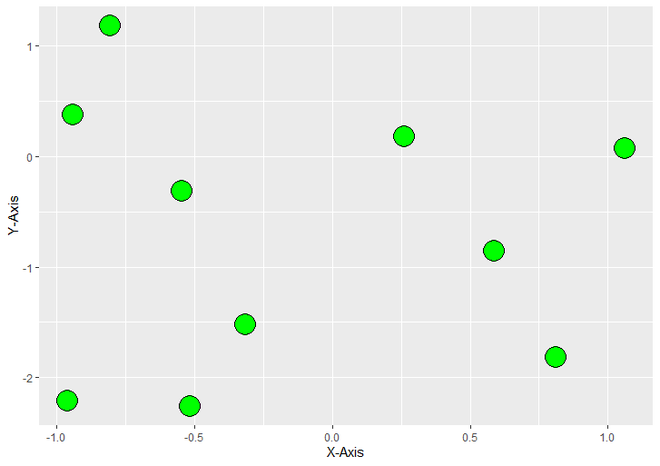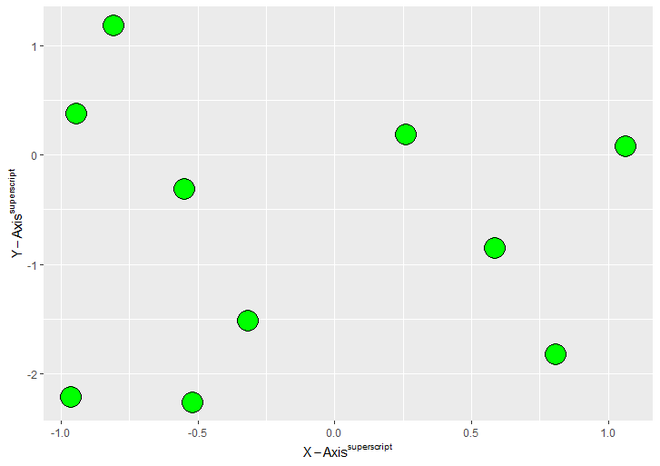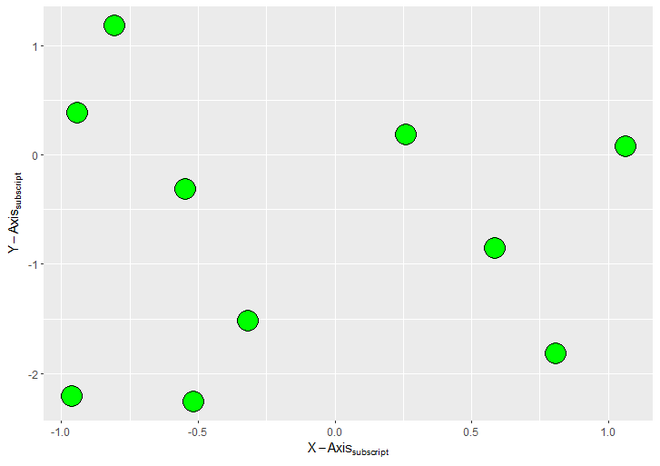# Superscript and subscript axis labels in ggplot2 in R

• Last Updated : 28 Sep, 2021

In this article, we will see how to use Superscript and Subscript axis labels in ggplot2 in R Programming Language.

First we should load ggplot2 package using library() function. To install and load the ggplot2 package, write following command to R Console.

```# To Install ggplot2 package
# (Write this command to R Console)
install.packages("ggplot2")

library("ggplot2")```

Now, let’s create a DataFrame. Here we will create a simple DataFrame with two variables named X & Y then assign it to the data object. Let’s named it DF. Here we have generated 10 random values for x and y axis using rnorm() function.

```# Load Package
library("ggplot2")

# Create a DataFrame
DF <- data.frame(X = rnorm(10),
Y = rnorm(10))```

To create an R plot, we use ggplot() function and for make it scattered we add geom_point() function to ggplot() function. Here we use some parameters size, fill, color, shape only for better appearance of points on ScatterPlot. For labels at X and Y axis, we use xlab() and ylab() functions respectively.

Syntax:

xlab(“Label for X-Axis”)

ylab(“Label for Y-Axis”)

Example:

## R

 `# Load Package``library``(``"ggplot2"``)`` ` `# Create a DataFrame ``DF <- ``data.frame``(X = ``rnorm``(10),                        ``                 ``Y = ``rnorm``(10))`` ` `# Create a ScatterPlot with simple labels``ggplot``(DF,``aes``(X, Y))+``  ``geom_point``(size = 8, fill = ``"green"``,``             ``color = ``"black"``, shape = 21)+``  ``xlab``(``"X-Axis"``)+``  ``ylab``(``"Y-Axis"``)`

Output:ScatterPlot with Simple Axis Labels

Now we will change the label of X to ” X-Axissuperscript ” and Y to ” Y-Axissuperscript “. For that bquote() function is used to quote the argument passed to it.

Syntax : bquote(expr)

Parameter :

• expr : language object

bquote() For SuperScript :

bquote(math superscript(^) Notation)

Example:

## R

 `# Load ggplot2 Package``library``(``"ggplot2"``)`` ` `# Create a DataFrame For Plotting``DF <- ``data.frame``(X = ``rnorm``(10),                        ``                 ``Y = ``rnorm``(10))`` ` `# Create ggplot2 ScatterPlot with SuperScripted ``# value of Label of Axis.``ggplot``(DF,``aes``(X, Y))+``  ``geom_point``(size = 8, fill = ``"green"``, ``             ``color = ``"black"``, shape = 21)+``  ``xlab``(``bquote``(X-Axis^superscript))+``  ``ylab``(``bquote``(Y-Axis^superscript))`

Output:ScatterPlot with Superscripted Axis Labels

We will change the label of X to ” X-Axissubscript ” and Y to “ Y-Axissubscript “. For that, we will again use the bquote() function but with different Mathematical Notation for subscript.

bquote() For Subscript :

bquote(math subscript([]) Notation)

Example:

## R

 `# Load ggplot2 Package``library``(``"ggplot2"``)`` ` `# Create a DataFrame For Plotting``DF <- ``data.frame``(X = ``rnorm``(10),                        ``                 ``Y = ``rnorm``(10))`` ` `# Create ggplot2 ScatterPlot with SubScripted ``# value of Label of Axis.``ggplot``(DF,``aes``(X, Y))+``  ``geom_point``(size = 8, fill = ``"green"``,``             ``color = ``"black"``, shape = 21)+``  ``xlab``(``bquote``(X-Axis[subscript]))+``  ``ylab``(``bquote``(Y-Axis[subscript]))`

Output:ScatterPlot with Subscripted Axis Labels

My Personal Notes arrow_drop_up